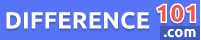Hyperbola

A hyperbola is a mathematical curve that is formed when a plane slices a double cone almost parallel to its axis. The resulting shape of the curve is symmetrical, with two separate branches that are identical in shape but facing different directions. The hyperbola is defined by two focal points, which are equidistant from the center of the curve, and the two axes of the curve – the transverse axis and the conjugate axis. The hyperbola can be found in many applications, including astronomy, physics, and engineering. Its properties and characteristics have been studied extensively by mathematicians over the centuries, making it an important part of the mathematics curriculum.